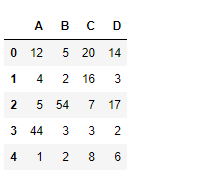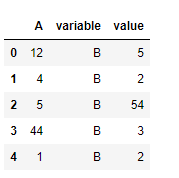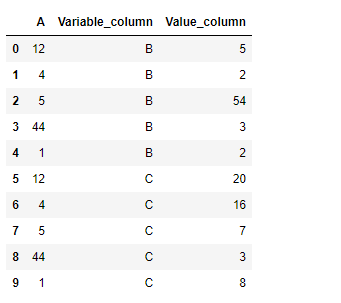# Python | Pandas dataframe.melt()

• Last Updated : 09 Dec, 2021

Python is a great language for doing data analysis, primarily because of the fantastic ecosystem of data-centric python packages. Pandas is one of those packages and makes importing and analyzing data much easier.

Pandas` dataframe.melt()` function unpivots a DataFrame from wide format to long format, optionally leaving identifier variables set. This function is useful to message a DataFrame into a format where one or more columns are identifier variables (id_vars), while all other columns, considered measured variables (value_vars), are “unpivoted” to the row axis, leaving just two non-identifier columns, ‘variable’ and ‘value’.

Syntax:DataFrame.melt(id_vars=None, value_vars=None, var_name=None, value_name=’value’, col_level=None)
Parameters :
frame : DataFrame
id_vars : Column(s) to use as identifier variables
value_vars : Column(s) to unpivot. If not specified, uses all columns that are not set as id_vars.
var_name : Name to use for the ‘variable’ column. If None it uses frame.columns.name or ‘variable’.
value_name : Name to use for the ‘value’ column
col_level : If columns are a MultiIndex then use this level to melt.

Returns: DataFrame into a format where one or more columns are identifier variables

Example #1: Use `melt()` function to set column “A” as the identifier variable and column “B” as value variable.

## Python3

 `# importing pandas as pd ` `import` `pandas as pd ` ` `  `# Creating the dataframe  ` `df ``=` `pd.DataFrame({``"A"``:[``12``, ``4``, ``5``, ``44``, ``1``],  ` `                   ``"B"``:[``5``, ``2``, ``54``, ``3``, ``2``],  ` `                   ``"C"``:[``20``, ``16``, ``7``, ``3``, ``8``],  ` `                   ``"D"``:[``14``, ``3``, ``17``, ``2``, ``6``]}) ` ` `  `# Print the dataframe ` `df `Lets use the `dataframe.melt()` function to set column “A” as identifier variable and column “B” as the value variable.

## Python3

 `# function to unpivot the dataframe ` `df.melt(id_vars ``=``[``'A'``], value_vars ``=``[``'B'``]) `

Output :Example #2: Use `melt()` function to set column “A” as the identifier variable and column “B” and “C” as value variable. Also customize the names of both the value and variable column.

## Python3

 `# importing pandas as pd ` `import` `pandas as pd ` ` `  `# Creating the dataframe  ` `df ``=` `pd.DataFrame({``"A"``:[``12``, ``4``, ``5``, ``44``, ``1``], ` `                   ``"B"``:[``5``, ``2``, ``54``, ``3``, ``2``],  ` `                   ``"C"``:[``20``, ``16``, ``7``, ``3``, ``8``],  ` `                   ``"D"``:[``14``, ``3``, ``17``, ``2``, ``6``]}) ` ` `  `# Print the dataframe ` `df `Lets use the `dataframe.melt()` function to set column “A” as identifier variable and column “B” and “C” as the value variable.

## Python3

 `# function to unpivot the dataframe ` `# We will also provide a customized name to the value and variable column ` ` `  `df.melt(id_vars ``=``[``'A'``], value_vars ``=``[``'B'``, ``'C'``],  ` `        ``var_name ``=``'Variable_column'``, value_name ``=``'Value_column'``) `

Output :My Personal Notes arrow_drop_up
Recommended Articles
Page :